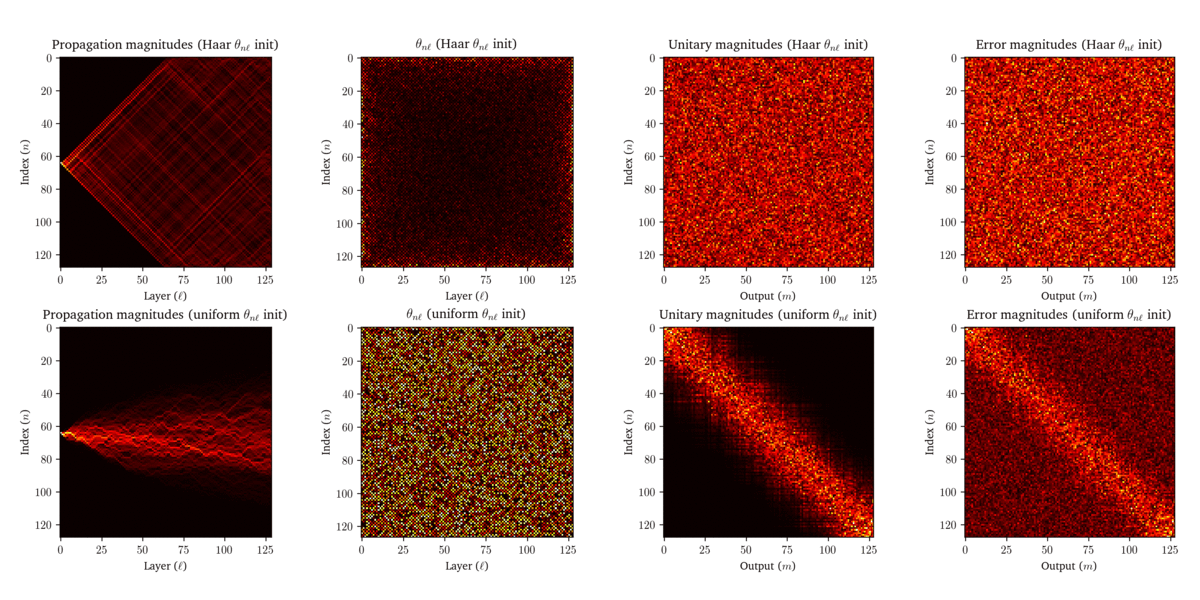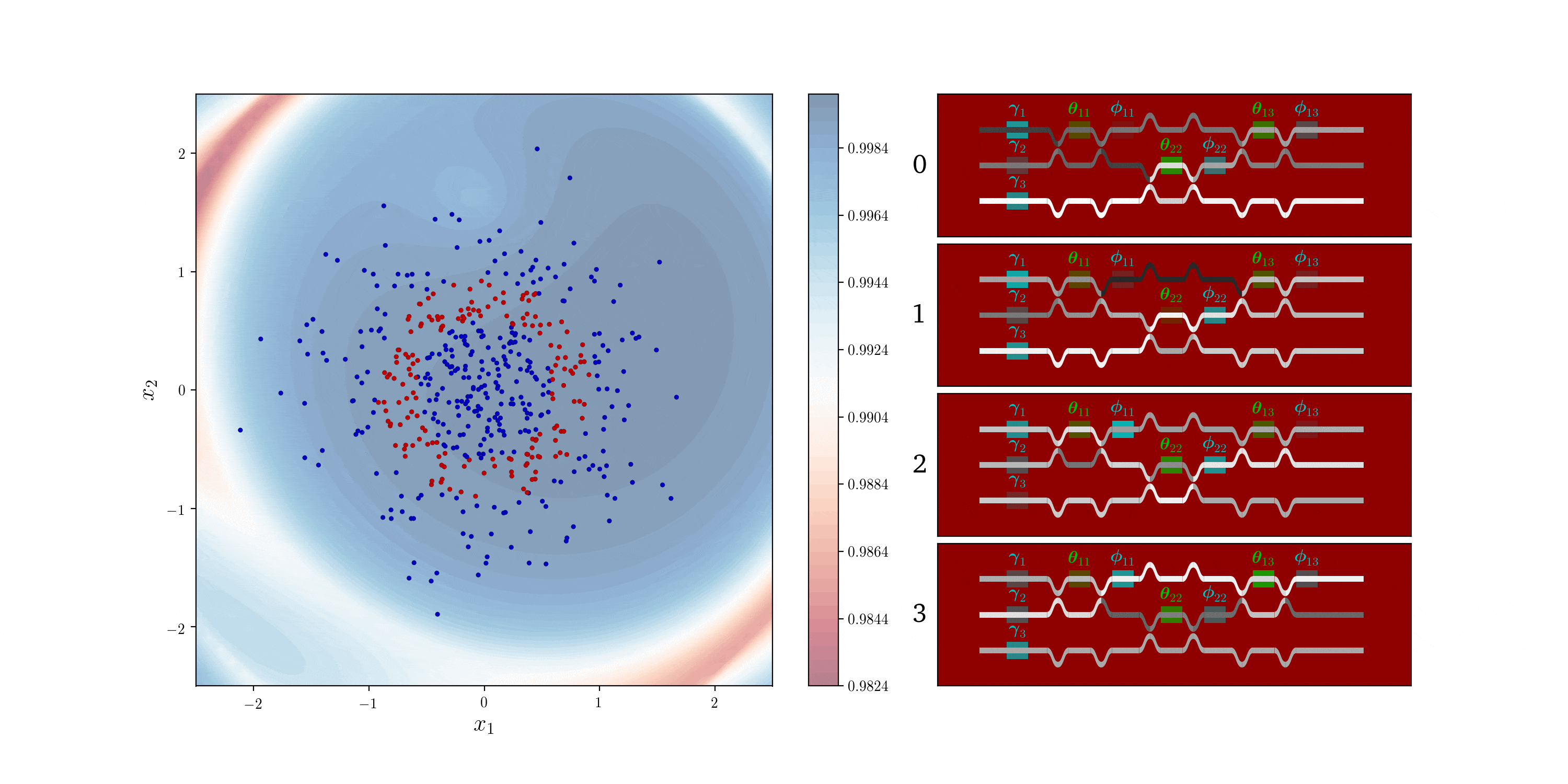{{ message }}

solgaardlab / neurophox Public

A simulation framework for unitary neural networks and photonic devices

Switch branches/tags
Nothing to show

Files

Failed to load latest commit information.
Type
Name
Commit time

The Neurophox module is an open source machine learning and photonic simulation framework based on unitary mesh networks presented in arxiv/1909.06179, arxiv/1808.00458, and arxiv/1903.04579.Motivation

Neurophox provides a robust and efficient framework for simulating optical neural networks (ONNs) that promise fast and energy-efficient machine learning. Scalable ONNs are made possible by integrated reconfigurable nanophotonic processors, feedforward networks of 2 x 2 nodes that compute matrix multiplication by simply allowing light to flow through them (thus, a time- and energy-efficient way to compute).

Reconfigurable photonic networks are currently being developed to scale in the optical domain using Mach-Zehnder interferometers (MZIs). Such matrix multiplier processors behave differently from conventional matrix multipliers:

1. They act as unitary operators rather than general linear operators, preserving the norm of the data flowing through the network (i.e., intensity of the light is preserved due to energy conservation).
2. The matrix elements are not directly trained during backpropagation. Instead, the node parameters are trained, and the matrix elements are nonlinear functions of those node parameters.

The goal of Neurophox is to provide utilities for calibrating and training such processors for machine learning and other signal processing tasks. These simulated models might furthermore be useful for a relatively unexplored regime of machine learning: "unitary machine learning" (see e.g., arxiv/1612.05231).

Dependencies and requirements

Some important requirements for Neurophox are:

1. Python >=3.6
2. Tensorflow >=2.0.0a
3. PyTorch

The dependencies for Neurophox (specified in requirements.txt) are:

numpy>=1.16
scipy
matplotlib
tensorflow>=2.0.0a

Optionally, the user may install torch>=1.7 to run the Pytorch layers. NOTE: PyTorch layers are currently nonfunctional as we migrate to complex PyTorch, but will be up and running once this issue is resolved.

Getting started

Installation

There are currently two options to install Neurophox:

1. Installation via pip:
pip install neurophox
2. Installation from source via pip:
git clone https://github.com/solgaardlab/neurophox
pip install -e neurophox
pip install -r neurophox/requirements.txt

If installing other dependencies manually, ensure you install PyTorch (since PyTorch mesh layers are currently in development) and Tensorflow 2.0.

Using the GPU

If using a GPU, we recommend using a conda environement to install GPU dependencies using CUDA 10.0 with the following commands:

conda install pytorch torchvision cudatoolkit=10.2 -c pytorch-nightly
pip install tensorflow-gpu==2.2.0

Features

Using Neurophox, we can simulate light physically propagating through such networks (using layer.propagate), and observe its equivalence to a matrix operation (using layer.matrix).

We demonstrate the Rectangular Mesh (RM layer) using either numpy or tensorflow:

import numpy as np
from neurophox.numpy import RMNumpy
from neurophox.tensorflow import RM

N = 16

tf_layer = RM(N)
np_layer = RMNumpy(N, phases=tf_layer.phases)

np.allclose(tf_layer.matrix, np_layer.matrix)  # True
np.allclose(tf_layer(np.eye(N)), np_layer.matrix)  # True

Visualizations

We provide code to visualize network phase shift parameters and light propagation in neurophox-notebooks for rectangular and triangular meshes.

For the phase shift settings above, we visualize the propagation of light (scaled by light intensity), equivalently representing data "flowing" through the mesh.

Small machine learning example

It is possible to compose Neurophox Tensorflow layers into unitary neural networks using tf.keras.Sequential to solve machine learning problems. Here we use absolute value nonlinearities and categorical cross entropy.

import tensorflow as tf
from neurophox.tensorflow import RM
from neurophox.ml.nonlinearities import cnorm, cnormsq

ring_model = tf.keras.Sequential([
RM(3, activation=tf.keras.layers.Activation(cnorm)),
RM(3, activation=tf.keras.layers.Activation(cnorm)),
RM(3, activation=tf.keras.layers.Activation(cnorm)),
RM(3, activation=tf.keras.layers.Activation(cnorm)),
tf.keras.layers.Activation(cnormsq),
tf.keras.layers.Lambda(lambda x: tf.math.real(x[:, :2])), # get first 2 output ports (we already know it is real from the activation),
tf.keras.layers.Activation('softmax')
])

ring_model.compile(
loss='categorical_crossentropy',
)

Below is a visualization for many planar classification problems:

Authors and citing this repository

Neurophox was written by Sunil Pai (email: sunilpai@stanford.edu).

If you find this repository useful, please cite at least one of the following papers depending on your application:

1. Definition and calibration of feedforward photonic networks:
@article{pai2019parallel,
title={Parallel fault-tolerant programming of an arbitrary feedforward photonic network},
author={Pai, Sunil and Williamson, Ian AD and Hughes, Tyler W and Minkov, Momchil and Solgaard, Olav and Fan, Shanhui and Miller, David AB},
journal={arXiv preprint arXiv:1909.06179},
year={2019}
}

2. Optimization of unitary mesh networks:
@article{pai_matrix_2019,
author = {Pai, Sunil and Bartlett, Ben and Solgaard, Olav and Miller, David A. B.},
doi = {10.1103/PhysRevApplied.11.064044},
journal = {Physical Review Applied},
month = jun,
number = {6},
pages = {064044},
title = {Matrix Optimization on Universal Unitary Photonic Devices},
volume = {11},
year = {2019}
}

3. Optical neural network nonlinearities:
@article{williamson_reprogrammable_2020,
author = {Williamson, I. A. D. and Hughes, T. W. and Minkov, M. and Bartlett, B. and Pai, S. and Fan, S.},
doi = {10.1109/JSTQE.2019.2930455},
issn = {1077-260X},
journal = {IEEE Journal of Selected Topics in Quantum Electronics},
month = jan,
number = {1},
pages = {1-12},
title = {Reprogrammable Electro-Optic Nonlinear Activation Functions for Optical Neural Networks},
volume = {26},
year = {2020}
}

Future Work and Contributions

Neurophox is under development and is not yet stable.

If you find a bug, have a question, or would like to recommend a feature, please submit an issue on Github.

We welcome pull requests and contributions from the broader community. If you would like to contribute, please submit a pull request and title your branch bug/bug-fix-title or feature/feature-title.

A simulation framework for unitary neural networks and photonic devices

5 tags

Packages 0

No packages published

•
•